# Cryptography: Theory and Practice:Secret Sharing Schemes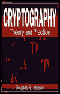Cryptography: Theory and Practice by Douglas Stinson CRC Press, CRC Press LLC ISBN: 0849385210   Pub Date: 03/17/95

What happens if a group of t - 1 participants attempt to compute K? Proceeding as above, they will obtain a system of t - 1 equations in t unknowns. Suppose they hypothesize a value y0 for the key. Since the key is a0 = a(0), this will yield a tth equation, and the coefficient matrix of the resulting system of t equations in t unknowns will again be a Vandermonde matrix. As before, there will be a unique solution. Hence, for every hypothesized value y0 of the key, there is a unique polynomial ay0(x) such that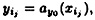1 ≤ jt - 1, and such that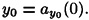Hence, no value of the key can be ruled out, and thus a group of t - 1 participants can obtain no information about the key.

We have analyzed the Shamir scheme from the point of view of solving systems of linear equations over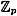. There is an alternative method, based on the Lagrange interpolation formula for polynomials. The Lagrange interpolation formula is an explicit formula for the (unique) polynomial a(x) of degree at most t that we computed above. The formula is as follows: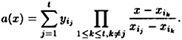It is easy to verify the correctness of this formula by substituting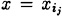: all terms in the summation vanish except for the jth term, which is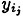. Thus, we have a polynomial of degree at most t - 1 which contains the t ordered pairs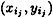, 1 ≤ jt. We already proved above that this polynomial is unique, so the interpolation formula does yield the correct polynomial.

A group B of t participants can compute a(x) by using the interpolation formula. But a simplification is possible, since the participants in B do not need to know the whole polynomial a(x). It is sufficient for them to compute the constant term K = a(0). Hence, they can compute the following expression, which is obtained by substituting x = 0 into the Lagrange interpolation formula: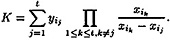Suppose we define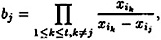1 ≤ jt. (Note that these values bj can be precomputed, if desired, and their values are not secret.) Then we have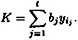Hence, the key is a linear combination of the t shares.

To illustrate this approach, let’s recompute the key from Example 11.1.

Example 11.1 (Con’t.)

The participants {P 1, P3, P5} can compute b1, b2, and b3 according to the formula given above. For example, they would obtain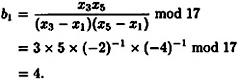Similarly, b2 = 3 and b3 = 11. Then, given shares 8, 10, and 11 (respectively), they would obtain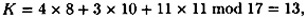as before.

The last topic of this section is a simplified construction for threshold schemes in the special case w = t. This construction will work for any key set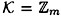with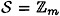. (For this scheme, it is not required that m be prime, and it is not necessary that mw + 1.) If D wants to share the key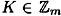, he carries out the protocol of Figure 11.2.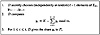Figure 11.2  A (t, t)-threshold scheme in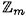Observe that the t participants can compute K by the formula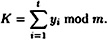Can t - 1 participants compute K? Clearly, the first t - 11 participants cannot do so, since they receive t - 1 independent random numbers as their shares. Consider the t - 1 participants in the set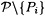, where 1 ≤ it - 1. These t - 1 participants possess the shares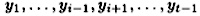and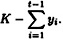By summing their shares, they can compute K - yi. However, they do not know the random value yi, and hence they have no information as to the value of K. Consequently, we have a (t, t)-threshold scheme.

### 11.2 Access Structures and General Secret Sharing

In the previous section, we desired that any t of the w participants should be able to determine the key. A more general situation is to specify exactly which subsets of participants should be able to determine the key and which should not. Let Γ be a set of subsets of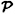; the subsets in Γ are those subsets of participants that should be able to compute the key. Γ is called an access structure and the subsets in Γ are called authorized subsets.

Let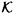be the key set and let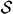be the share set. As before, when a dealer D wants to share a key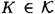, he will give each participant a share from. At a later time a subset of participants will attempt to determine K from the shares they collectively hold.

DEFINITION 11.2  A perfect secret sharing scheme realizing the access structure Γ is a method of sharing a key K among a set of w participants (denoted by), in such a way that the following two properties are satisfied:

1.  If an authorized subset of participants,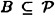pool their shares, then they can determine the value of K.
2.  If an unauthorized subset of participantspool their shares, then they can determine nothing about the value of K.

Observe that a (t, w)-threshold scheme realizes the access structure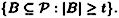Such an access structure is called a threshold access structure. We showed in the previous section that the Shamir scheme is a perfect scheme realizing the threshold access structure.

We study the unconditional security of secret sharing schemes. That is, we do not place any limit on the amount of computation that can be performed by an unauthorized subset of participants.

Suppose that B ∈ Γ and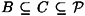. Suppose the subset C wants to determine K. Since B is an authorized subset, it can already determine K. Hence, the subset C can determine K by ignoring the shares of the participants in C\B. Stated another way, a superset of an authorized set is again an authorized set. What this says is that the access structure should satisfy the monotone property: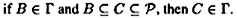In the remainder of this chapter, we will assume that all access structures are monotone.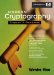Modern Cryptography: Theory and Practice
ISBN: 0130669431
EAN: 2147483647
Year: 1995
Pages: 133
Authors: Wenbo Mao

Similar book on Amazon